( ) round brackets; parentheses { } curly brackets; braces [ ] square brackets; brackets a = b a equals b; or a is equal to b a ≠ b a is not equal to b a > b a is greater than b a2 > ad a second is greater than a dth b < a b is less than a a >> b a is substantially greater than b a ≥ b a is greater than or equal to b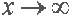x trends to infinity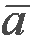a vector ; the mean volume of athe first derivative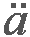the second derivative 9.510 nine thousand five hundred and ten 32 + 8 = 40 thirty-two plus eight is (are) forty; or, thirty-two plus eight equals forty; or, thirty-two plus eight is equal to forty; or, eight added to thirty-two makes forty 20 - 5 = 15 twenty minus five is fifteen; or, twenty minus five is equal to (equals) fifteen; or, twenty minus five leaves fifteen; or, five from twenty is (leaves) fifteen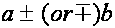a plus or minus b 1 × 1 = 1 once one is one 2 × 2 = 4 twice two is (equals) four; or, twice two makes four 6 × 10 = 60 six multiplied by ten equals sixty; or, six multiplied by ten is (equal to) sixty; or, six times ten is sixty work = force × distance work is (equal' to) the product of the force multiplied by the distance; or, work is (equal to) the product of force times the distance 12 : 3 = 4 twelve divided by three equals (is) four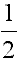a (one) half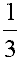a (one) third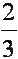two-thirdsfive-ninths 4½ four and a half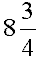eight and three-quarters 0.6 or .6 point six 5.34 five point thirty-four; or, five point three four 2.01 two point nought one; or two point o [ou] one 0.007 point nought nought seven; or, point two oes [ouz] seven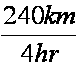240 kilometers pro 4 hours 8 : 4 = 2 the ratio of eight to four is two. 20 : 5 = 16 : 4 or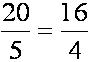the ratio of twenty to five equals (is equal to) the ratio of sixteen to four; or, twenty is to five as sixteen is to four 20° twenty degrees 6´ six minutes; also, six f eet 10´´ ten seconds; also, ten inches a´ a prime a´´ a second prime; or a double prime; or a twice dashed a´´´ a triple prime 92 nine square, or, the square of nine or, nine to the second power 63 six cubed; or, six to the third (power) c18 c [si:] to the eighteenth (power) a-10 a [ei] to the minus tenth (power)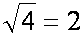the square root (out) of four is (equals) two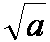the square root of a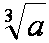the cube root of a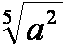the fifth root of a squarea plus b all squared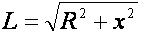L equals the square root (out) of R square plus x square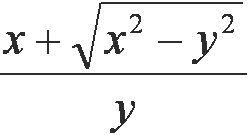x plus square root of x square minus y square all over y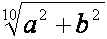the tenth root (out) of a square plus b square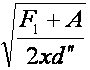square root out of F first plus A divided by two xd th twice dashed (or double prime)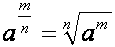a to the m/n th power equals the nth root out of a to the mth (power)dz over dx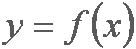y is a function of x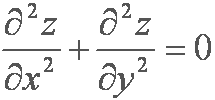partial d two z over partial dx plus partial d two z over partial dy equals zero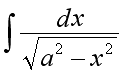indefinite integral of dx (divided) by the square root out of a2 minus x2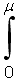integral from zero to µ (mu)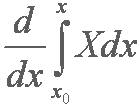d (divided) by dx (or d over dx) of the integral from x nought to x of X large dx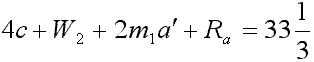4c plus W second plus 2 m first a prime plus R ath equals thirty-three and one-third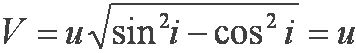V equals u square root of sine square i minus cosine square i equals utangent r equals tangent i divided by l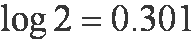the logarithm of two equals zero point three o[ou] one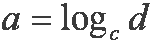a is equal to the logarithm of d to the base c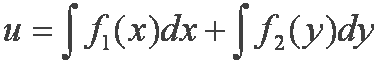u is equal to the integral of f sub one of x multiplied by dx plus the integral of f sub two of y multiplied by dyK is equal to the maximum over j of the sum from i equals one to i equals n of the modulus of aij of t , where t lies in the closed interval ab and where j runs from one to n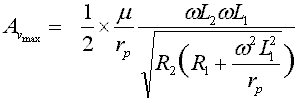A vmax is equal to one half mu by r pth omega L second omega L first (divided) by square root out of R second round brackets opened R first plus omega square L first square by r pth round brackets closed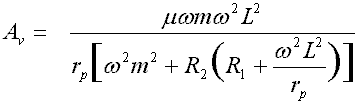Av is equal to mu omega m omega square L square (divided) by r pth square brackets opened omega square m square plus R second round brackets opened R first plus omega square L square (divided) by r pth round and square brackets closed